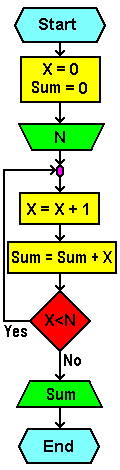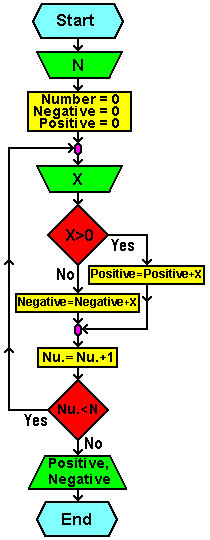## Example IXWrite an algorithm and flowchart for the addition of the first N integers.

Algorithm:

```start
-----------------------------------------------------
X=0
SUM = 0
Input N
X = X + 1
SUM = SUM + X
If X < N
Then go back to 'X = X + 1'
Print SUM
-----------------------------------------------------
end
```
 Flowchart: Program code:``` -------------------------------------- 10 CLS 20 CLEAR 30 X = 0: sum = 0 40 INPUT "Input aggregate limit:" N DO X = X + 1 sum = sum + X LOOP WHILE X < N REM Using 'LOOP UNTIL' at first REM complied the condition REM goes out of the loop 50 PRINT "Sum is:" sum 60 END -------------------------------------- 10 CLS 20 CLEAR 30 x = 0: sum = 0 40 INPUT "Input aggregate limit:" N FOR i = 1 TO N STEP 1 x = x + 1 sum = sum + x NEXT i 50 PRINT "Sum is:" sum 60 END -------------------------------------- ```## Example X

Write an algorithm and flowchart that loads N integers. Calculate the sum of all positive and the sum of all negative numbers.

Algorithm:

```start
-----------------------------------------------------
Input N
number = 0
positive = 0
negative = 0
Input X
If X > 0
Then positive = positive + X
Else negative = negative + x
number = number + 1
If number < N
Then go back to 'Input X'
Print positive, negative
-----------------------------------------------------
end
```
 Flowchart: Program code:``` 10 CLS 20 CLEAR 30 number = 0 40 positive = 0 50 negative = 0 60 INPUT "Input aggregate limit:" N DO INPUT "Input larger of limit:" X IF X > 0 THEN positive = positive + X ELSE negative = negative + X END IF number = number + 1 LOOP WHILE X < N REM Using 'LOOP UNTIL' at first REM complied the condition REM goes out of the loop 70 PRINT "Sum of positive:" positive 80 PRINT "Sum of negative:" negative 90 END ```Citing of this page: Radic, Drago. " IT - Informatics Alphabet " Split-Croatia. {Date of access}. . Copyright © by Drago Radic. All rights reserved. | Disclaimer